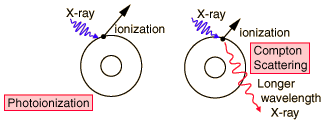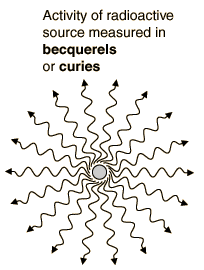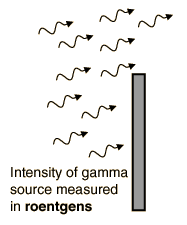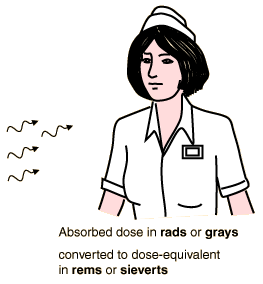Because the energies of the particles emitted during radioactive processes are extremely high, nearly all such particles fall in the class of ionizing radiation.Activity of source Absorbed dose Biologically effective dose Intensity Old standard unit Curie Rad Rem Roentgen SI unit Becquerel Gray Sievert ...
Index

 HyperPhysics***** Nuclear R Nave
Go Back

The practical threshold for radiation risk is that of ionization of tissue. Since the ionization energy of a hydrogen atom is 13.6 eV, the level around 10 eV is an approximate threshold. Since the energies associated with nuclear radiation are many orders of magnitude above this threshold, in the MeV range, then all nuclear radiation is ionizing radiation. Likewise, x-rays are ionizing radiation, as is the upper end of the ultraviolet range.

 All nuclear radiation must be considered to be ionizing radiation! In addition, the upper end of the electromagnetic spectrum is ionizing radiation.Index

 HyperPhysics***** Nuclear R Nave
Go Back

The curie (Ci) is the old standard unit for measuring the activity of a given radioactive sample. It is equivalent to the activity of 1 gram of radium. It is formally defined by:1 curie = amount of material that will produce 3.7 x 1010 nuclear decays per second. 1 becquerel = amount of material which will produce 1 nuclear decay per second. 1 curie = 3.7 x 1010 becquerels. The bequerel is the more recent SI unit for radioactive source activity.

Index

 HyperPhysics***** Nuclear R Nave
Go Back

The roentgen (R) is a measure of radiation intensity of xrays or gamma rays. It is formally defined as the radiation intensity required to produce an ionization charge of 0.000258 coulombs per kilogram of air. It is one of the standard units for radiation dosimetry, but is not applicable to alpha, beta, or other particle emission and does not accurately predict the tissue effects of gamma rays of extremely high energies. The roentgen has mainly been used for calibration of xray machines.Index

 HyperPhysics***** Nuclear R Nave
Go Back

 The rad is a unit of absorbed radiation dose in terms of the energy actually deposited in the tissue. The rad is defined as an absorbed dose of 0.01 joules of energy per kilogram of tissue. The more recent SI unit is the gray, which is defined as 1 joule of deposited energy per kilogram of tissue. To assess the risk of radiation, the absorbed dose is multiplied by the relative biological effectiveness of the radiation to get the biological dose equivalent in rems or sieverts.Index

 HyperPhysics***** Nuclear R Nave
Go Back

Biologically Effective Dose

 The biologically effective dose in rems is the radiation dose in rads multiplied by a "quality factor" which is an assessment of the effectiveness of that particular type and energy of radiation. For alpha particles the relative biological effectiveness (rbe) may be as high as 20, so that one rad is equivalent to 20 rems. However, for x-rays and gamma rays, the rbe is taken as one so that the rad and rem are equivalent for those radiation sources. The sievert is equal to 100 rems.